# 老張讓我用TensorFlow識別語音命令：前進、停止、左轉、右轉

## 二、我的研究

### 2.1 語音的解析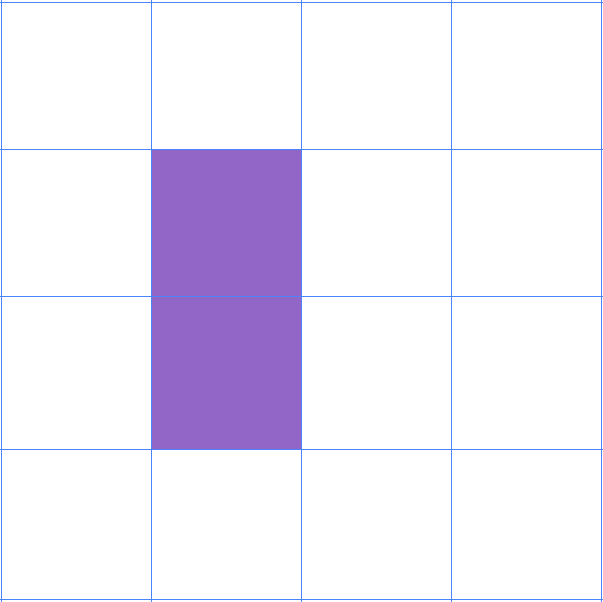``` python

# 根據文件路徑，解碼音頻文件

import tensorflow as tf audio_binary = tf.io.read_file("datasets\go\1000.wav") audio, rate = tf.audio.decode_wav(contents=audio_binary) ```

```python import matplotlib.pyplot as plt plt.plot(audio) plt.show()```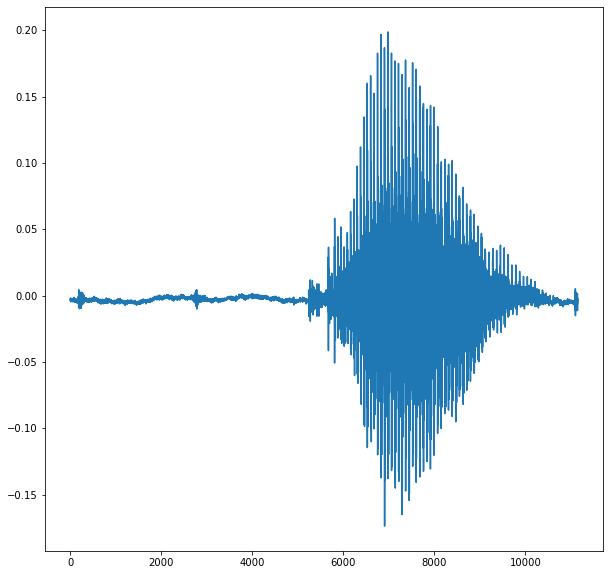```python import matplotlib.pyplot as plt plt.plot(audio[0:10]) plt.show()```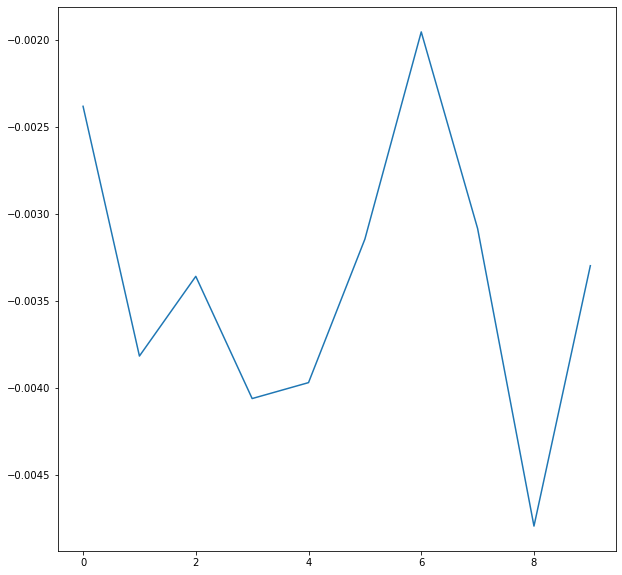### 2.2 音頻的頻譜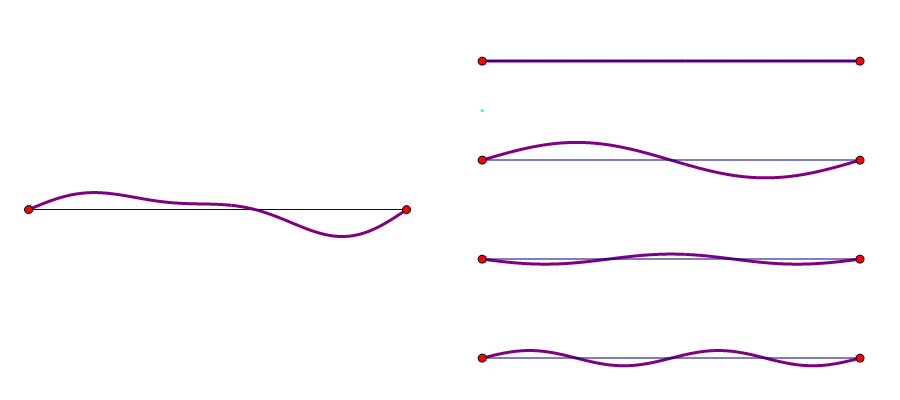```python waveform = tf.squeeze(audio, axis=-1) spectrogram = tf.signal.stft(waveform, frame_length=255, frame_step=128)```

`tf.signal.stft`裏面的參數，是指取小樣的規則。就是從總波形裏面，每隔多久取多少小樣本進行分析。分析之後，我們也是可以像繪製波形一樣，把分析的頻譜結果繪製出來的。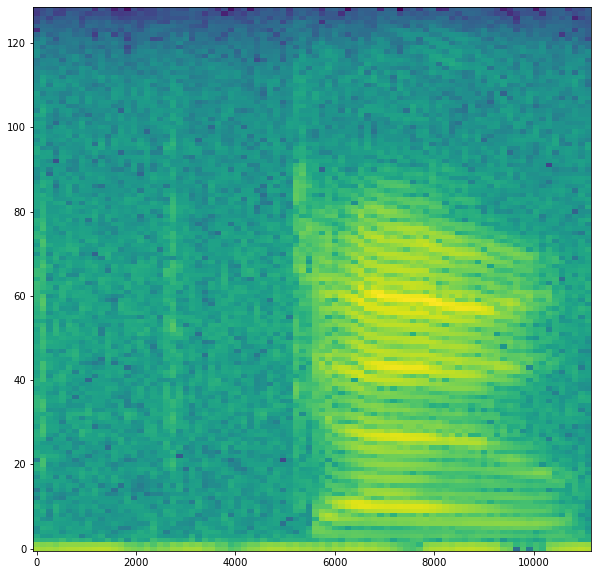### 2.3 音頻數據的預處理```牀前明月光〇〇 一頓不吃餓得慌 蜀道難〇〇〇〇```

``` <tf.Tensor: shape=(86, 129, 1), dtype=float32, numpy= array([[[4.62073803e-01], ..., [2.92062759e-05]],

``````   [[3.96062881e-01],
[2.01166332e-01],
[2.09505502e-02],
...,
[1.43915415e-04]]], dtype=float32)>
``````

```

```python waveform = tf.squeeze(audio, axis=-1) input_len = 16000 waveform = waveform[:input_len] zero_padding = tf.zeros( - tf.shape(waveform),dtype=tf.float32) waveform = tf.cast(waveform, dtype=tf.float32) equal_length = tf.concat([waveform, zero_padding], 0) spectrogram = tf.signal.stft(equal_length, frame_length=255, frame_step=128) spectrogram = tf.abs(spectrogram) spectrogram = spectrogram[..., tf.newaxis]```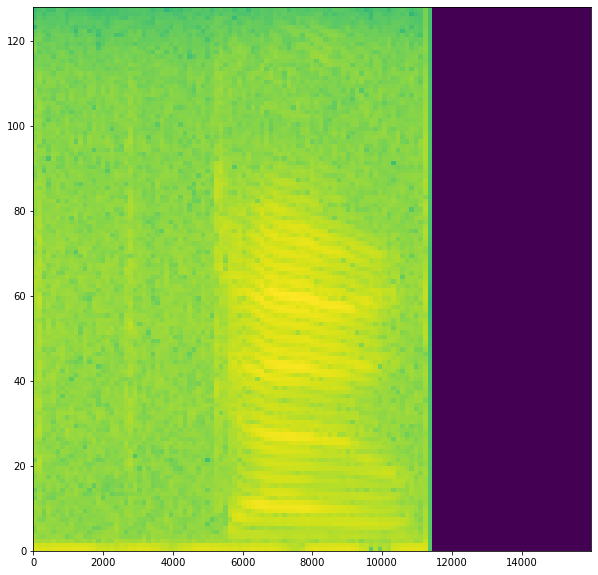### 2.4 構建模型和訓練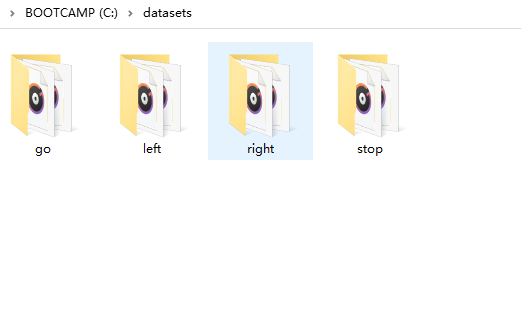``` python import tensorflow as tf from tensorflow.keras import layers from tensorflow.keras import models

model = models.Sequential([ layers.Input(shape= (124, 129, 1)), layers.Resizing(32, 32), layers.Normalization(), layers.Conv2D(32, 3, activation='relu'), layers.Conv2D(64, 3, activation='relu'), layers.MaxPooling2D(), layers.Dropout(0.25), layers.Flatten(), layers.Dense(128, activation='relu'), layers.Dropout(0.5), layers.Dense(4), ]) model.compile( optimizer=tf.keras.optimizers.Adam(), loss=tf.keras.losses.SparseCategoricalCrossentropy(from_logits=True), metrics=['accuracy'] ) ```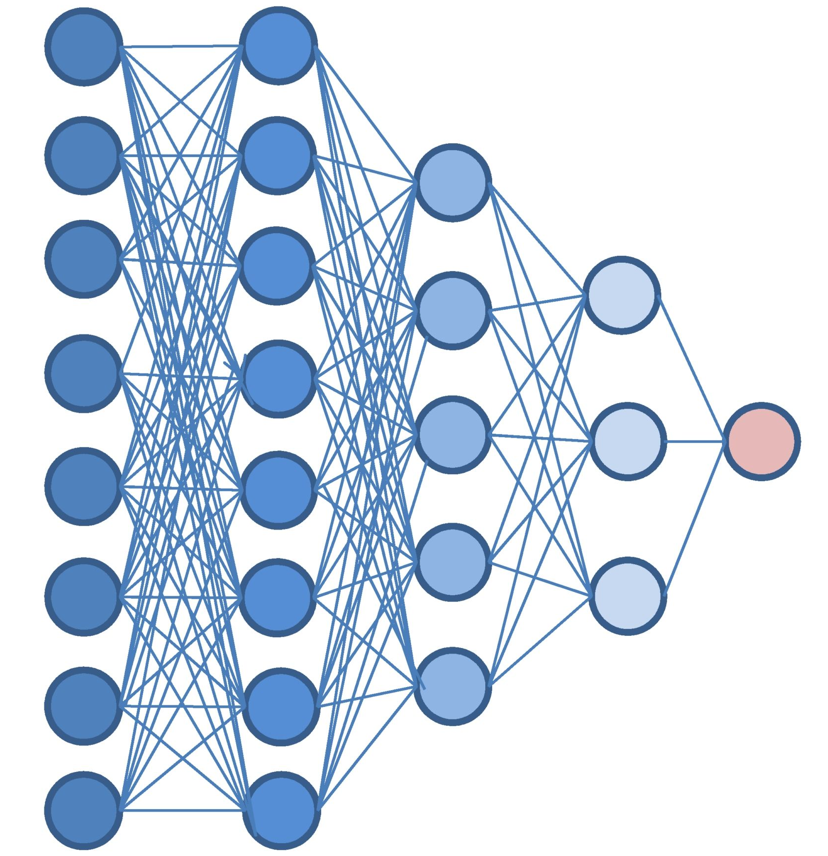```python model = create_model() cp_callback = tf.keras.callbacks.ModelCheckpoint(filepath='model/model.ckpt', save_weights_only=True, save_best_only=True) history = model.fit( train_ds, validation_data=val_ds, epochs=50, callbacks=[cp_callback] )```

`filepath='model/model.ckpt'`表示訓練完成後，存儲的路徑。`save_weights_only=True`只存儲權重數據。`save_best_only=True`意思是隻存儲最好的訓練的結果。調用訓練很簡單，調用`model.fit`，傳入訓練集、驗證集、訓練輪數、以及訓練回調就可以啦。

### 2.5 加載模型並預測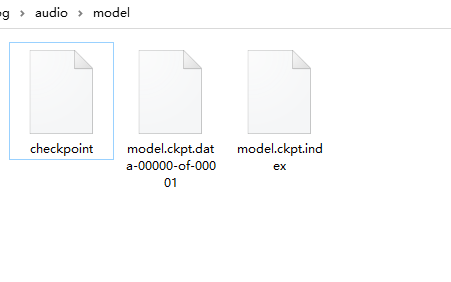``` python model = create_model() if os.path.exists('model/model.ckpt.index'): model.load_weights('model/model.ckpt')
labels = ['go', 'left', 'right', 'stop']

# 音頻文件轉碼

audio = get_audio_data('mysound.wav') audios = np.array([audio]) predictions = model(audios) index = np.argmax(predictions) print(labels[index]) ```

「其他文章」